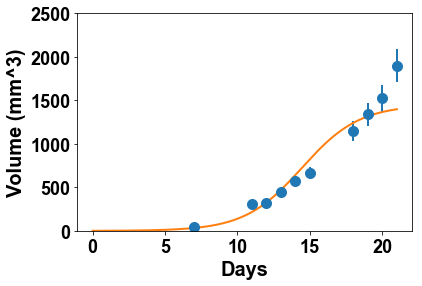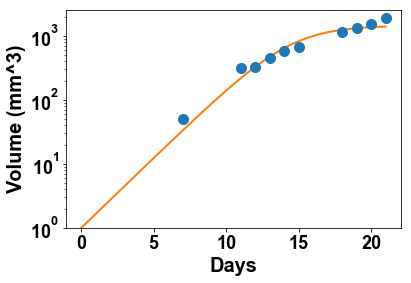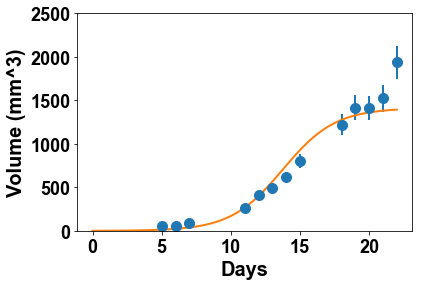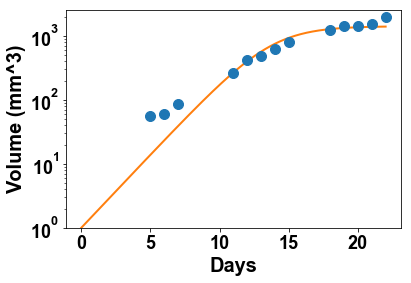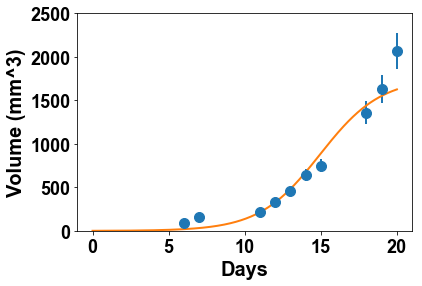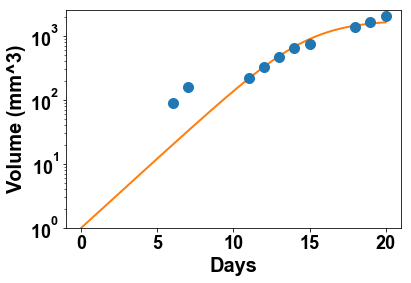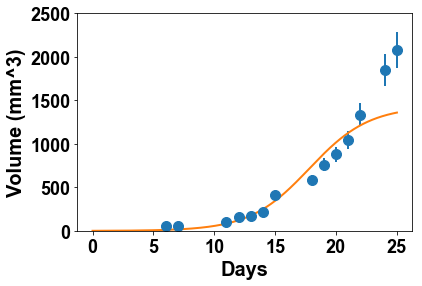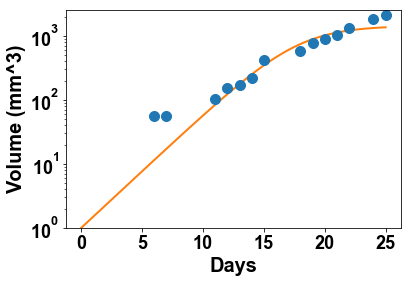# 2. Tumor growth laws¶

Problem:

Considering $n$ volume observations $(V_1,\cdots,V_n)$ at time points $(t_1, \cdots, t_n)$, we would like to see whether these data could have been generated by a given function $$V: \begin{array}{ccc} \mathbb{R}\times \mathbb{R}^p & \longrightarrow & \mathbb{R} \\ (t,\theta) & \longmapsto & V(t,\theta)\end{array}$$ depending on time $t$ and a vector of parameters $\theta\in \mathbb{R}^p$.

Another – closely related – problem is to find a set of parameters $\hat{\theta}$ that would "best" describe the data.

In our context a model will be the combination of two parts:

1. The structural model $V$ (deterministic)
2. An observation model linking the model to the observations (error model, stochastic)

The latter is defined by assuming that the observations were generated by the model plus some measurements error and we write $$V_j = V(t_j; \theta) + \sigma_j \varepsilon_j.$$ where $\sigma_j\varepsilon_j$ describes the error term. It is natural to assume that this error term is gaussian, with expectation zero. $\sigma_j$ is the standard deviation of the error and the $\varepsilon_j$ are independent and identically distributed random variables with $$\varepsilon_j \sim \mathcal{N}(0, 1).$$

Error model

An important assumption of the model is how does $\sigma_j$ depend on the volume. The first step would be to consider $\sigma_j$ as constant (i.e. independent of $j$), which would mean that for all the observations, the magnitude of the error is the same. However, it appears also reasonable to consider that from our measurement technique (calipers), larger tumors would result in larger errors. A natural assumption could then be a proportional error model described by $\sigma_j = \sigma V(t_j, \theta)$. In our case, a detailed study of 133 measurements that were performed twice on the same tumor established a slightly more refined model but we will assume a proportional error model for simplicity.

Likelihood maximization

A central concept in model fitting and parameter estimation is the notion of likelihood and likelihood maximization. The likelihood of the data is defined as the probability density function of the data, under the assumption it has been generated by the model $V$ with parameters $\theta$ and $\sigma$. Note that $\sigma$ is often unknown and is a parameter to be established. However in our analysis we could compute it from the analysis mentioned above and we will take here $\sigma = 0.1$ (10% error). From the formula above, under the independence and normality assumption we can compute it to get $$L(\theta) = p(V_1,\cdots,V_n;\theta)=\prod_{j=1}^n p(V_j ;\theta) = \prod_{j=1}^n \frac{1}{\sigma_j\sqrt{2 \pi}}e^{-\frac{\left(V_j - V(t_j,\theta)\right)^2}{2\sigma_j^2}}$$ At this point, it is natural to consider the log-likelihood $l(\theta, \sigma)=\ln\left(L(\theta)\right)$ to simplify the calculations. We get \begin{align} l(\theta) & = - \sum_{j= 1}^{n} \frac{\left(V_j - V(t_j,\theta)\right)^2}{2\sigma_j^2} - \sum_{j=1}^n \ln(\sigma_j) - n \ln(\sqrt{2\pi}) \\ l(\theta) & = - \sum_{j= 1}^{n} \frac{\left(V_j - V(t_j,\theta)\right)^2}{2\sigma^2 V(t_j, \theta)^2} - n \ln(\sigma) - \sum_{j=1}^n \ln(V(t_j,\theta)) - n \ln(\sqrt{2\pi}) \end{align} To simplify further, we will replace $V(t_j, \theta)$ by the observation $V_j$ in the terms above coming from the error model (third term and denominator in the first sum). The maximization of the log-likelihood then becomes equivalent to the following weighted least squares minimization problem (the $\sigma$s can be removed because they don't change the minimization problem): $$\hat{\theta} = \underset{\theta}{\rm argmin}\left|\left|\frac{V-V(t;\theta)}{V}\right|\right|_2^2.$$ where $V = (V_1, \cdots, V_n)$, $V(t;\theta) = (V(t_1; \theta), \cdots, V(t_n; \theta))$ and $||\cdot||_2$ is the discrete $L^2$ norm (sum of squares).

Import modules and load the data

In :
% matplotlib inline
%precision %.3g

Out:
'%.3g'
In :
import numpy as np
import pandas as pd
import matplotlib.pyplot as plt

In :
df   = pd.read_excel('data_table.xlsx')
time = df.index.values


## 2.1 Proliferation and the exponential model¶

We will first explore the validity of the exponential growth model to test what was observed by plotting the curves. The model is defined by a constant length of the cell cycle (thus a constant doubling time) for a constant fraction of the tumor volume. In equations, this translates into $$\left\lbrace\begin{array}{l} \frac{dV}{dt} = \alpha V\\ V(t=0) = V_0 \end{array} \right. \quad \Longrightarrow \quad V(t) = V_0 e^{\alpha t}$$ where $V_0$ is the initial volume and $\alpha$ is the proliferation rate, defined by $\alpha=\frac{\ln(2)}{\tau}$ with $\tau$ the length of the cell cycle.

Define a python function exponential_V0 taking a time vector time and a vector of parameters param as input (in this order) and giving the exponential model value as output.

In :
def exponential_V0(time, alpha, V0):
V     = V0*np.exp(alpha*time)
return V


By manual exploration of the parameters and plotting the first mouse growth data together with model simulations, test different values of the parameters that would best describe the data

In :
alpha = 0.2
V0    = 20
V     = exponential_V0(time, alpha, V0)
plt.errorbar(df.index, df, 0.1*df, fmt='o')
plt.plot(time, V)

Out:
[<matplotlib.lines.Line2D at 0x118e1c518>]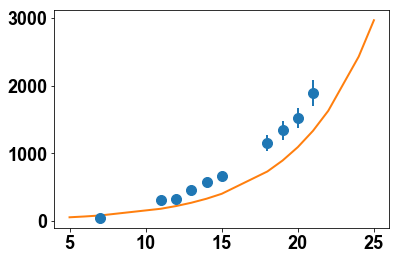Using the function curve_fit from the module scipy.optimize, find the parameters $\hat{\alpha}^1$ and $\hat{V_0}^1$ that best fit the data of the first mouse.

In :
from scipy.optimize import curve_fit


To get the data vector with no nan and corresponding time vector, use (example with $j=1$)

In :
time_1 = time[~np.isnan(df)]
data_1 = df[~np.isnan(df)]

In :
popt, pcov = curve_fit(exponential_V0, time_1, data_1, [alpha, V0], sigma=0.1*data_1)
print('alpha_opt = %.3g' %popt)
print('V0_opt = %.3g' %popt)

alpha_opt = 0.248
V0_opt = 12.4


Plot the resulting curve and data (with 10% error bar). Plot the model curve from day 0. What would you conclude in terms of the validity of the model?

In :
time_plot = np.linspace(0, time_1[-1], 1000)
V_plot    = exponential_V0(time_plot, popt, popt)
plt.errorbar(df.index, df, 0.1*df, fmt='o')
plt.plot(time_plot, V_plot)
plt.xlabel('Days')
plt.ylabel('Volume (mm^3)')

Out:
Text(0,0.5,'Volume (mm^3)')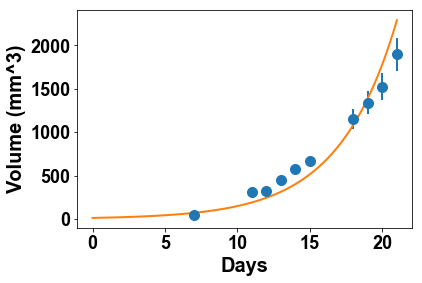Plot the curve in log scale with plt.yscale('log') and set the limits of the y axis to (1, 2500) with plt.ylim(1, 2500). Remembering that at day 0 $10^6$ cells (equivalent to 1 mm$^3$) were injected in the animal, what do you think of the plausibility of the model for the entire duration of the experiment?

In :
plt.figure(2)
plt.errorbar(df.index, df, 0.1*df, fmt='o')
plt.plot(time_plot, V_plot)
plt.xlabel('Days')
plt.ylabel('Volume (mm^3)')
plt.yscale('log')
plt.ylim(1, 2500)

Out:
(1, 2500)
/Users/benzekry/anaconda3/lib/python3.6/site-packages/matplotlib/scale.py:111: RuntimeWarning: invalid value encountered in less_equal
out[a <= 0] = -1000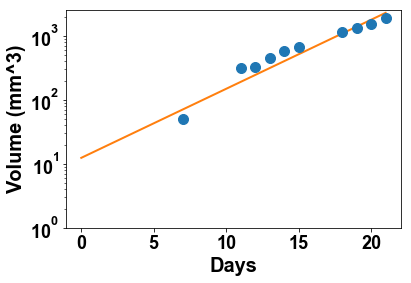Repeat this for all the mice (use a for loop) but plot only in log scale. What do you conclude from the values of $V_0$ obtained and the plots?

In :
for mouse in range(1, 11):
time_loc   = time[~np.isnan(df[mouse])]
data_loc   = df[mouse][~np.isnan(df[mouse])]
popt, pcov = curve_fit(exponential_V0, time_loc, data_loc, [alpha, V0], sigma=0.1*data_loc)
print('alpha_opt = %.3g' %popt)
print('V0_opt = %.3g' %popt)
V_plot     = exponential_V0(time_plot, popt, popt)
plt.figure(mouse)
plt.errorbar(df.index, df[mouse], 0.1*df[mouse], fmt='o')
plt.plot(time_plot, V_plot)
plt.xlabel('Days')
plt.ylabel('Volume (mm^3)')
plt.yscale('log')
plt.ylim(1, 2500)

alpha_opt = 0.248
V0_opt = 12.4
alpha_opt = 0.215
V0_opt = 20.8
alpha_opt = 0.223
V0_opt = 24.2
alpha_opt = 0.208
V0_opt = 12.9
alpha_opt = 0.222
V0_opt = 16.8
alpha_opt = 0.27
V0_opt = 10.3
alpha_opt = 0.26
V0_opt = 20.5
alpha_opt = 0.226
V0_opt = 18
alpha_opt = 0.273
V0_opt = 5.37
alpha_opt = 0.238
V0_opt = 9

/Users/benzekry/anaconda3/lib/python3.6/site-packages/matplotlib/scale.py:111: RuntimeWarning: invalid value encountered in less_equal
out[a <= 0] = -1000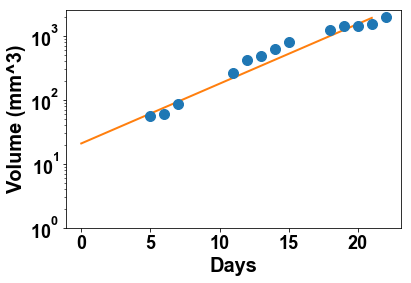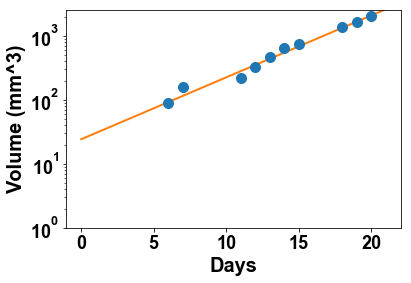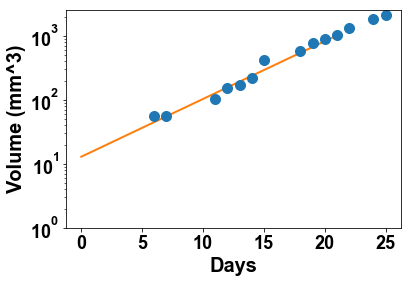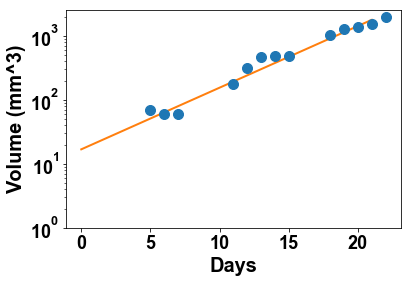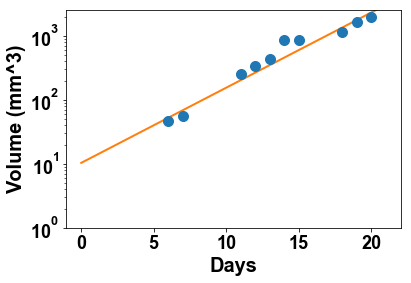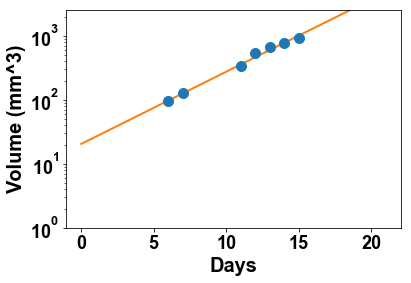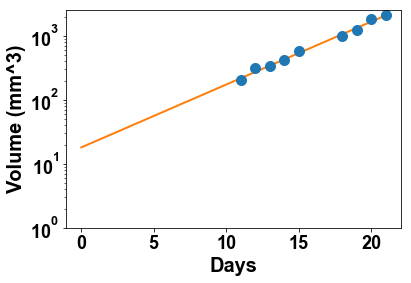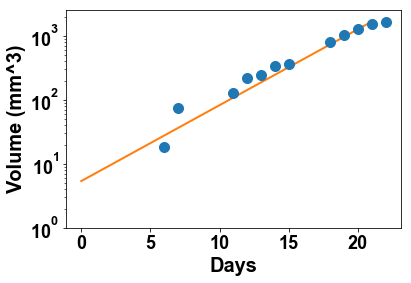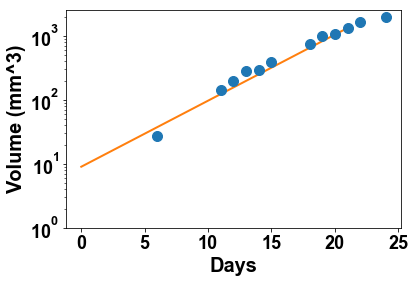To test this further, let's use the information on the number of injected cells as an initial condition and define another model, named exponential defined by $$\left\lbrace\begin{array}{l} \frac{dV}{dt} = \alpha V\\ V(t=0) = 1 \end{array} \right. \quad \Longrightarrow \quad V(t) = e^{\alpha t}$$

In :
def exponential(time, alpha):
return np.exp(alpha*time)

In :
for mouse in range(1, 11):
time_loc   = time[~np.isnan(df[mouse])]
data_loc   = df[mouse][~np.isnan(df[mouse])]
popt, pcov = curve_fit(exponential, time_loc, data_loc, alpha, sigma=0.1*data_loc)
print('alpha_opt = %.3g' %popt)
V_plot     = exponential(time_plot, popt)
plt.figure(2*mouse)
plt.errorbar(df.index, df[mouse], 0.1*df[mouse], fmt='o')
plt.plot(time_plot, V_plot)
plt.xlabel('Days')
plt.ylabel('Volume (mm^3)')
plt.ylim(1, 2500)
plt.figure(2*mouse+1)
plt.errorbar(df.index, df[mouse], 0.1*df[mouse], fmt='o')
plt.plot(time_plot, V_plot)
plt.xlabel('Days')
plt.ylabel('Volume (mm^3)')
plt.yscale('log')
plt.ylim(1, 2500)

alpha_opt = 0.377
alpha_opt = 0.361
alpha_opt = 0.399
alpha_opt = 0.324
alpha_opt = 0.362
alpha_opt = 0.397
alpha_opt = 0.479
alpha_opt = 0.38
alpha_opt = 0.358
alpha_opt = 0.338

/Users/benzekry/anaconda3/lib/python3.6/site-packages/matplotlib/scale.py:111: RuntimeWarning: invalid value encountered in less_equal
out[a <= 0] = -1000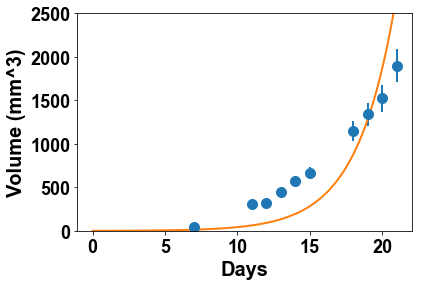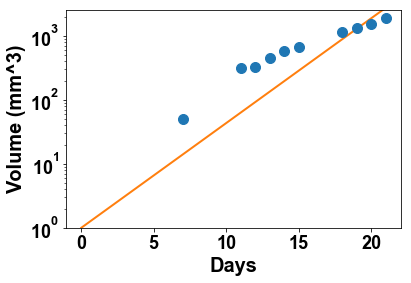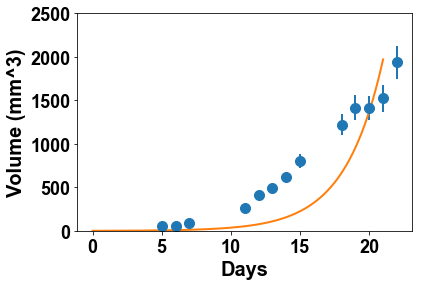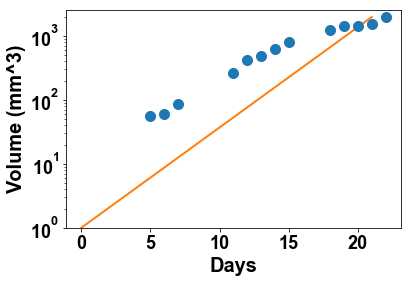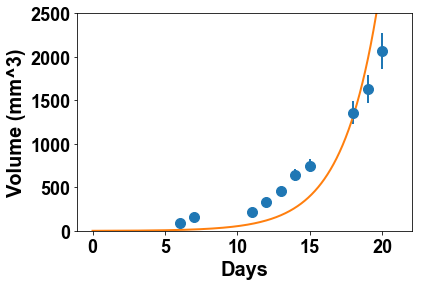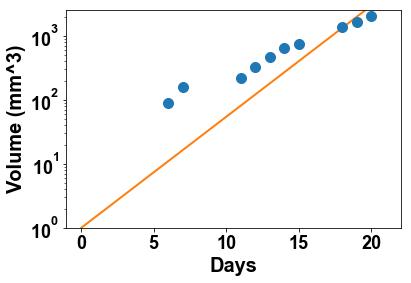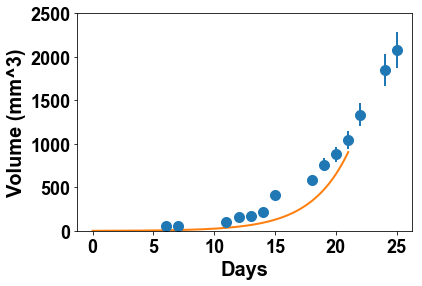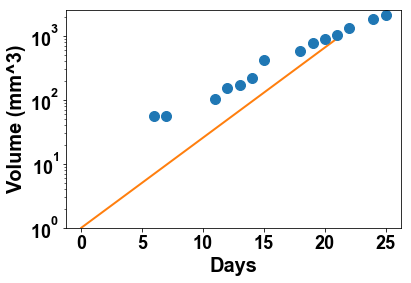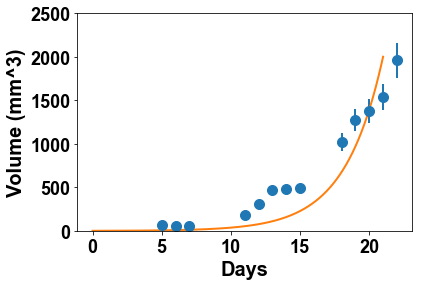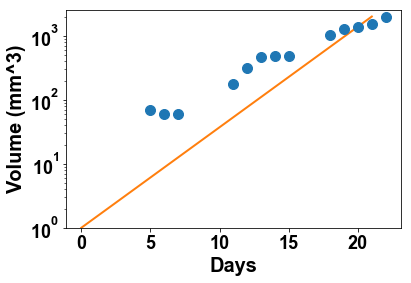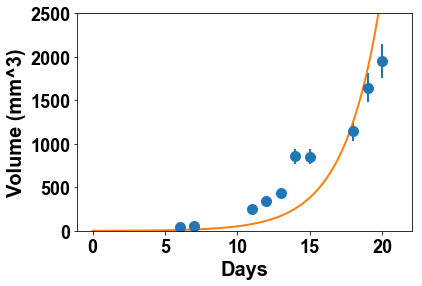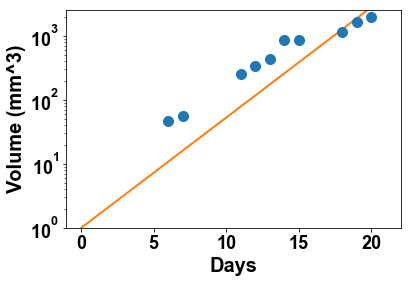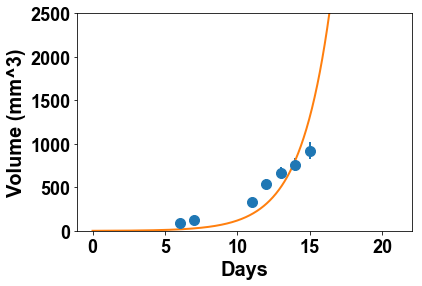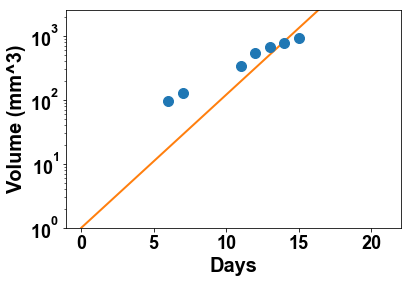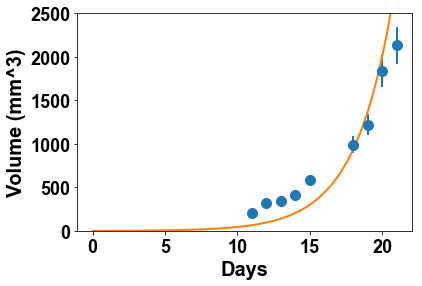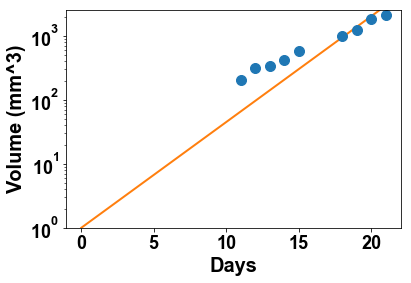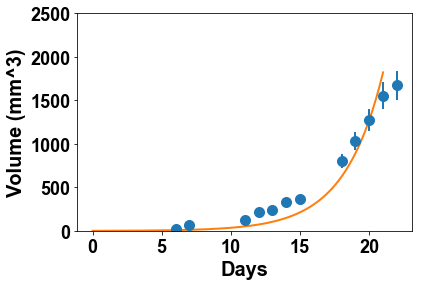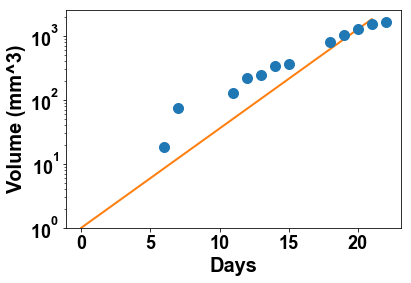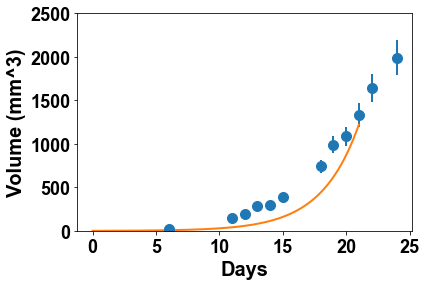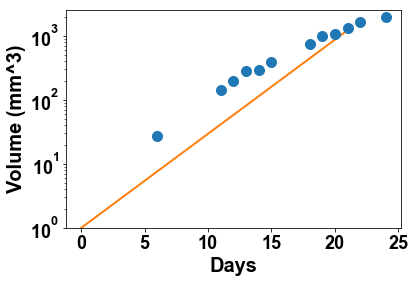## 2.2 Competition and the logistic model¶

The above observations demonstrated that the tumor growth had to be faster than their actual growth rate during the observation phase, if starting from $V(t=0) = 1$ mm$^3$. This suggests to look for models that would exhibit such growth deceleration. In ecology, when modeling the growth of a population, a famous model for explaining growth deceleration and saturation is the logistic model. A tumor being a population of cells, it appears natural to test this model against our data. The logistic model states that the individuals (here the tumor cells) would compete for nutrients or space. Introducing the concept of carrying capacity $K$ as the maximal reachable size for the population, the fraction of cells able to divide is then $1-\frac{V}{K}$ and the model writes $$\left\lbrace\begin{array}{l} \frac{dV}{dt} = \alpha V\left(1 - \frac{V}{K}\right)\\ V(t=0) = 1 \end{array} \right. \quad \Longrightarrow \quad V(t) = \frac{K}{1+(K-1)e^{-\alpha t}}.$$

Define a python function logistic for simulation of this model

In :
def logistic(time, alpha, K):
V = K/(1+(K-1)*np.exp(-alpha*time))
return V


Define a function fit_all_mice that takes as input a model function and initial parameters, fits the model to the 10 individual tumor growth kinetics and plots these fits both in arithmetic and logarithmic scale. Set the option maxfev=10000 when calling curve_fit.

In :
def fit_all_mice(model_f, param0):
for mouse in range(1, 11):
time_loc   = time[~np.isnan(df[mouse])]
data_loc   = df[mouse][~np.isnan(df[mouse])]
popt, pcov = curve_fit(model_f, time_loc, data_loc, param0, sigma=0.1*data_loc, maxfev=10000)
print(popt)
time_plot  = np.linspace(0, time_loc[-1], 1000)
V_plot     = model_f(time_plot, *popt)
plt.figure(2*mouse)
plt.errorbar(df.index, df[mouse], 0.1*df[mouse], fmt='o')
plt.plot(time_plot, V_plot)
plt.xlabel('Days')
plt.ylabel('Volume (mm^3)')
plt.ylim(1, 2500)
plt.figure(2*mouse+1)
plt.errorbar(df.index, df[mouse], 0.1*df[mouse], fmt='o')
plt.plot(time_plot, V_plot)
plt.xlabel('Days')
plt.ylabel('Volume (mm^3)')
plt.yscale('log')
plt.ylim(1, 2500)


Apply it to the logistic model with initial parameters $\alpha = 0.5$ and $K = 5000$. What do you think of the visual accuracy of the fits? Comment also on the plausibility of the inferred values of $K$.

In :
alpha = 0.5
K     = 5000
fit_all_mice(logistic, [alpha, K])

[  5.03860131e-01   1.44964365e+03]
[  5.27244972e-01   1.41192700e+03]
[  4.99815175e-01   1.75627985e+03]
[  4.08117450e-01   1.43174842e+03]
[  4.84841337e-01   1.39187850e+03]
[  5.17437787e-01   1.52860872e+03]
[   0.72430748  588.50815217]
[  4.65311047e-01   1.66158324e+03]
[  4.37049763e-01   1.50147207e+03]
[  4.34415244e-01   1.47095044e+03]

/Users/benzekry/anaconda3/lib/python3.6/site-packages/matplotlib/scale.py:111: RuntimeWarning: invalid value encountered in less_equal
out[a <= 0] = -1000